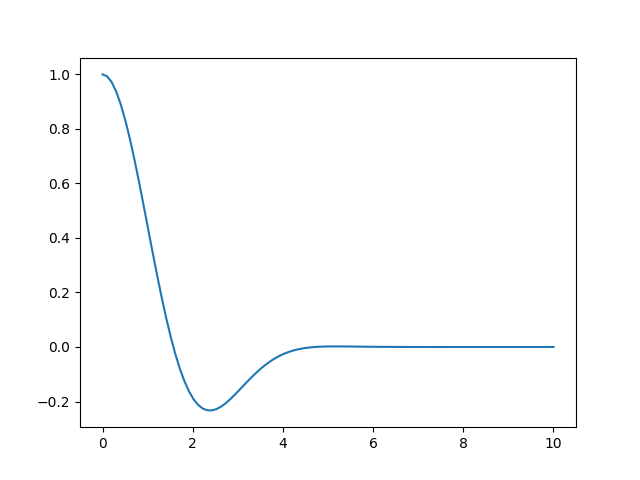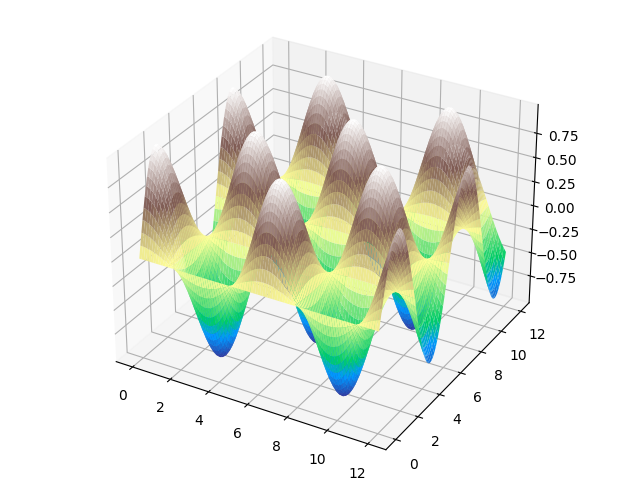a field-theory motivated approach to computer algebra

# cdb.graphics.plot

Plotting of functions
Functionality to make plots of functions.
import numpy as np import matplotlib.pyplot as plt

## plot(ex: Ex, range: tuple) -> plot

Plot a function of one variable given the range.
The function should be a Cadabra expression (so written using dollar symbols if it is constructed inline). The range is a tuple or list with three elements: the symbol which is the dependent variable as a Cadabra expression, the starting value and the end value.
plot($\cos(x)*\exp(-x**2/5)$, ($x$, 0, 10));{}$\big[$$\big]$

## plot3d(ex: Ex, range1: tuple, range2: tuple) -> plot

Plot a function of two variables given their ranges.
The function should be a Cadabra expression (so written using dollar symbols if it is constructed inline). The ranges is are tuples with three elements each: the symbol which is the dependent variable as a Cadabra expression, the starting value and the end value.
plt.clf() plot3d( $\sin(x)\cos(y)$, ($x$, 0, 12), ($y$, 0, 12));